>  Chemistry Test - 9

# Chemistry Test - 9

Test Description

## 45 Questions MCQ Test | Chemistry Test - 9

 1 Crore+ students have signed up on EduRev. Have you?
Chemistry Test - 9 - Question 1

### Which is high spin complex ?

Detailed Solution for Chemistry Test - 9 - Question 1

Only complex [Cr(gly)3​] is a high spin complex because it contains a weak field ligand and these type of ligands can not pair up the unpaired electrons while in other options there are atleast  one strong field ligand. Hence,option A is correct.

Chemistry Test - 9 - Question 2

### Ligands in a complex salt are

Chemistry Test - 9 - Question 3

### Correct formula of the complex formed in the brown ring test for nitrates is

Chemistry Test - 9 - Question 4

A complex cation is formed by Pt (in same oxidation state) with ligands (in proper number so that coordination number of Pt becomes six). Which of the following can be its correct IUPAC name ?

Chemistry Test - 9 - Question 5

Which of the following is incorrect reaction ?

Chemistry Test - 9 - Question 6

The magnetic moment of a transition metal ion is found to be 3.87 B.M. The number of unpaired electrons present in it is

Chemistry Test - 9 - Question 7

Cl2 gas is dried over

Chemistry Test - 9 - Question 8

Which one of the following pentafluorides cannot be formed ?

Chemistry Test - 9 - Question 9

Which of the following statements is incorrect ?

Chemistry Test - 9 - Question 10

Which one is monobasic acid ?

Chemistry Test - 9 - Question 11

The oxidation state of sulphur in the anions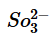,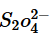,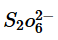follows the order

Chemistry Test - 9 - Question 12

Four metals and their methods of refinement are given
I. Ni, Cu, Zr, Ga
II. electrolysis, van Arkel process, zone refining, Mond’s process
Choose the right method for each

Chemistry Test - 9 - Question 13

Which of the following chemicals is used as a depressant in separating ZnS from PbS in froth floatation process ?

Chemistry Test - 9 - Question 14

Which of the isomeric C4H9Cl compounds will be optically active ?

Chemistry Test - 9 - Question 15

The relative ease of dehydration of following alcohols is
I. CH3CH(OH)CH3
II. (CH3)3COH  III. CH3CH2CH2OH

Chemistry Test - 9 - Question 16

Correct arrangement of the following acids in decreasing order of pKa values is
I. CH3COOH     II. Cl2CHCOOH
III. F3CCOOH   IV. ClCH2COOH
V. FCH2COOH

Chemistry Test - 9 - Question 17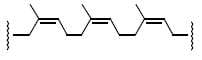The given polymer is

Chemistry Test - 9 - Question 18

Arrange the following
I. CH3CH2CH2Cl
II. CH3CH2CH(Cl)CH3
III. (CH3)2CHCH2Cl
IV. (CH3)3CCl
in order of decreasing tendency towards SN2 reaction

Chemistry Test - 9 - Question 19

Which one of the following reaction is a method for the conversion of a ketone into a hydrocarbon ?

Chemistry Test - 9 - Question 20

The reagent which does not react with both acetone and benzaldehyde is

Chemistry Test - 9 - Question 21

Hinsenberg’s reagent is

Chemistry Test - 9 - Question 22

The van’t Hoff factor for a 0.1 M Al2(SO4)3 solution is 4.20. The degree of dissociation is

Chemistry Test - 9 - Question 23

The product (Z) of the following sequence of reaction is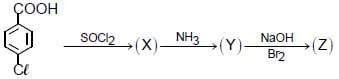Chemistry Test - 9 - Question 24

Match List I with List II and select the correct answer using the codes given below the lists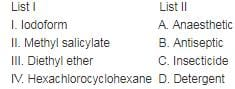Chemistry Test - 9 - Question 25

Such detergent is formed when stearic acid reacts with polytthene glycol.

Chemistry Test - 9 - Question 26

Unit of frequency factor A in k = Ae-Ea/RT  is

Chemistry Test - 9 - Question 27

In which case should N2(g) be more soluble in water?

Chemistry Test - 9 - Question 28

Which of the following does not undergo HVZ reaction ?

Chemistry Test - 9 - Question 29

The two monosaccharides of sucrose are held together by a glycosidic linkage between

Chemistry Test - 9 - Question 30

When ammonia gas is brought in contact with water surface, its pressure falls due to

Chemistry Test - 9 - Question 31

When a solution of AgNO3 (1 M) is electrolysed using platinum anode and copper cathode.What are the products obtained at two electrodes ?
Given :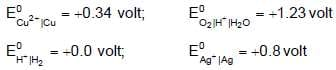Chemistry Test - 9 - Question 32

If the radii of A+ and B- in the crystalline solid AB are 96 pm and 200 pm respectively. The type of voids occupied is

Chemistry Test - 9 - Question 33

Select the incorrect statement among the following,.

Chemistry Test - 9 - Question 34

Which of the following factors is responsible for the decrease in the rate of a surface catalysed reaction?

Chemistry Test - 9 - Question 35

A quantity of iodine was reacted with a large excess of propanone and dilute acid. The equation for the reaction was

I2 + CH3COCH3 → ICH2COCH3 + HI

The concentration of iodine in the reaction mixture was recorded at regular time intervals and the result is shown in figure. Which one the following deductions can be made from this graph ?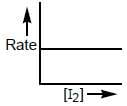Chemistry Test - 9 - Question 36

The equivalent conductance at infinite dilution of a weak acid such as HF

Chemistry Test - 9 - Question 37

A sample of element has 12.08 x 1023 bcc unit cells. Total number of atoms in the given sample are

Chemistry Test - 9 - Question 38

Equivalent conductivity of Fe2(SO4)3 is related to molar conductivity by the expression

Chemistry Test - 9 - Question 39

(R) in the given reaction sequence is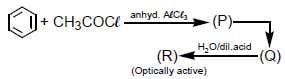Chemistry Test - 9 - Question 40

Which of the following alcohols will yield the corresponding alkyl chloride on reaction with concentrated HCl at room temperature ?

Chemistry Test - 9 - Question 41

Which of the following has ester linkage ?

Chemistry Test - 9 - Question 42

A polymer of prop-2-ene nitrile is called

Chemistry Test - 9 - Question 43(Scan QR code)